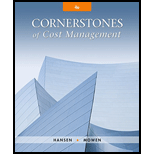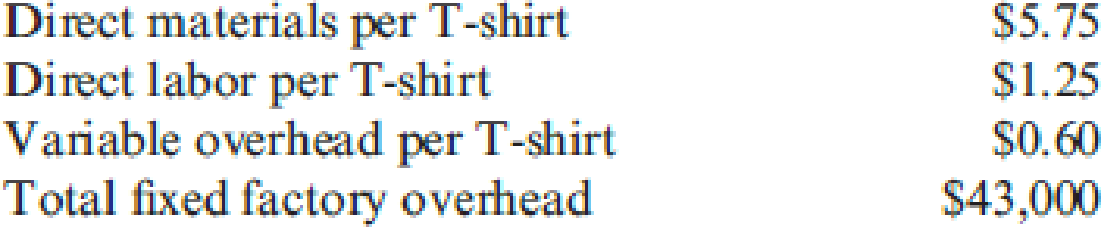# Super-Tees Company plans to sell 12,000 T-shirts at $16 each in the coming year. Product costs include: Variable selling expense is the redemption of a coupon, which averages$0.80 per T-shirt; fixed selling and administrative expenses total $19,000. Required: 1. Calculate the: a. Variable product cost per unit b. Total variable cost per unit c. Contribution margin per unit d. Contribution margin ratio (rounded to four significant digits) e. Total fixed expense for the year 2. Prepare a contribution-margin-based income statement for Super-Tees Company for the coming year. 3. What if the per unit selling expense increased from$0.80 to $1.75? Calculate the new values for the following: a. Variable product cost per unit b. Total variable cost per unit c. Contribution margin per unit d. Contribution margin ratio (rounded to four significant digits) e. Total fixed expense for the yearBuyFindarrow_forward ### Cornerstones of Cost Management (C... 4th Edition Don R. Hansen + 1 other Publisher: Cengage Learning ISBN: 9781305970663 #### Solutions Chapter SectionBuyFindarrow_forward ### Cornerstones of Cost Management (C... 4th Edition Don R. Hansen + 1 other Publisher: Cengage Learning ISBN: 9781305970663 Chapter 16, Problem 1CE Textbook Problem 109 views ## Super-Tees Company plans to sell 12,000 T-shirts at$16 each in the coming year. Product costs include:Variable selling expense is the redemption of a coupon, which averages $0.80 per T-shirt; fixed selling and administrative expenses total$19,000.Required: 1. Calculate the: a. Variable product cost per unit b. Total variable cost per unit c. Contribution margin per unit d. Contribution margin ratio (rounded to four significant digits) e. Total fixed expense for the year 2. Prepare a contribution-margin-based income statement for Super-Tees Company for the coming year. 3. What if the per unit selling expense increased from $0.80 to$1.75? Calculate the new values for the following: a. Variable product cost per unit b. Total variable cost per unit c. Contribution margin per unit d. Contribution margin ratio (rounded to four significant digits) e. Total fixed expense for the year

1. a.

To determine

Compute the variable product cost per unit.

### Explanation of Solution

Variable Cost: Variable costs refer to the costs that are involved in the production, and vary as per the changes in the volume of production.

Compute the variable product cost per unit of T-shirt:

Variable Product Cost=Direct materials + Direct labor +�

1. b.

To determine

Compute the total variable cost per unit.

1. c.

To determine

Compute the contribution margin cost per unit.

1. d.

To determine

Compute the contribution margin ratio.

1. e.

To determine

Compute the total fixed expense for the year.

2.

To determine

Draw up the contribution margin based income statement for Company ST.

3. a.

To determine

Compute the variable product cost per unit.

3. b.

To determine

Compute the total variable cost per unit.

3. c.

To determine

Compute the contribution margin cost per unit.

3. d.

To determine

Compute the contribution margin ratio.

3. e.

To determine

Compute the total fixed expense for the year.

### Still sussing out bartleby?

Check out a sample textbook solution.

See a sample solution

#### The Solution to Your Study Problems

Bartleby provides explanations to thousands of textbook problems written by our experts, many with advanced degrees!

Get Started

Find more solutions based on key concepts
Identify the five major steps in the accounting cycle.

Intermediate Accounting: Reporting And Analysis

Describe marketing uses of branding

MKTG 12:STUDENT ED.-TEXT

Two investors are evaluating GEs stock for possible purchase. They agree on the expected value of D1 and on the...

Fundamentals of Financial Management, Concise Edition (with Thomson ONE - Business School Edition, 1 term (6 months) Printed Access Card) (MindTap Course List)

What is a digital envelope?

Accounting Information Systems

List the five functions of an MIS.

Foundations of Business (MindTap Course List)